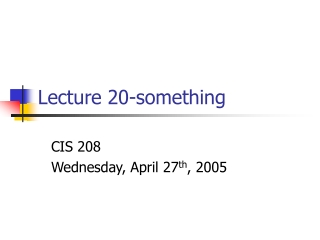DownloadDownload PresentationLecture 20-something

# Lecture 20-something

Télécharger la présentation## Lecture 20-something

- - - - - - - - - - - - - - - - - - - - - - - - - - - E N D - - - - - - - - - - - - - - - - - - - - - - - - - - -
##### Presentation Transcript

1. Lecture 20-something CIS 208 Wednesday, April 27th, 2005

2. Announcements • Friday is last day of class • Final homework is assigned • Due in two weeks

3. Final Assignment • Build an electronic address book. • Use either C or C++ • May work in pairs.

4. Final HW • Due Wednesday of final’s week, by noon. • Turn in source code and a manual or readme file. • Tell me about the different features.

5. Object Orientated design • Hiding implementation • Let’s the user not care about how things work. • Primary purpose of OO design.

6. OO Design • Give objects most of the functionality • Give the user as many choices as possible. • Main programs should be simple.

7. References • Call-by-reference without pointers • Lets the user forget about pointers. • Accessed without pointer notion. • CBR only.

8. references. • A swap function void swap(int *p1, int *p2) { int temp = *p1; *p1 = *p2; *p2 = temp; } int a = 1, b =2; swap(&a,&b);

9. Now easier void swap(int &p1, int &p2){ int temp = p1; p1 = p2; p2 = temp; } int a = 1, b= 2; swap(a,b); • Don’t need to use * or & anymore • Call by value is replaced with call by reference

10. Some issues • Can’t change where reference is pointing. • Passing object references doesn’t call deconstructor • Can’t reference a reference • Can’t create arrays of references • Must be initialized.

11. Returning references char &replace(int i); //return a reference char s = “Hello there”; int main() { replace(5) = ‘X’; cout << s; return 0; } char &replace(int i) { return s[i]; }

12. Independent references int main() { int a = 10; int &ref = a; ref = 100; cout << a << “ “<< ref; int b = 19; ref = b; cout <<a << “ “ << ref; ref--; cout << a << “ “ << ref; return 0; }

13. derived references • base reference can point to derived object. • Not the other way around.